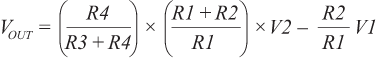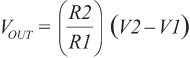# A Deeper Look into Difference Amplifiers

### Introduction

The classic four-resistor difference amplifier seems simple, but many circuit implementations perform poorly. Based on actual production designs, this article shows some of the pitfalls encountered with discrete resistors, filtering, ac common-mode rejection, and high noise gain.

College electronics courses illustrate applications for ideal op amps, including inverting and noninverting amplifiers. These are then combined to create a difference amplifier. The classic four resistor difference amplifier, shown in Figure 1, is quite useful and has been described in textbooks and literature for more than 40 years.

The transfer function of this amplifier is(1)

With R1 = R3 and R2 = R4, Equation 1 simplifies to(2)

This simplification occurs in textbooks, but never in real life, as the resistors are never exactly equal. In addition, other modifications of the basic circuit can yield unexpected behavior. The following examples come from real application questions, although they have been simplified to show the essence of the problem.

### CMRR

An important function of the difference amplifier is to reject signals that are common to both inputs. Referring to Figure 1, if V2 is 5 V and V1 is 3 V, for example, then 4 V is common to both. V2 is 1 V higher than the common voltage, and V1 is 1 V lower. The difference is 2 V, so the "ideal" gain of R2/R1 would be applied to 2 V. If the resistors are not perfect, part of the common-mode voltage will be amplified by the difference amplifier and appear at VOUT as a valid difference between V1 and V2 that cannot be distinguished from a real signal. The ability of the difference amplifier to reject this is called common-mode rejection (CMR). This can be expressed as a ratio (CMRR) or converted to decibels (dB).

In a 1991 article, Ramón Pallás-Areny and John Webster showed that the common-mode rejection, assuming a perfect op amp, is(3)

where Ad is the gain of the difference amplifier and t is the resistor tolerance. Thus, with unity gain and 1% resistors, the CMRR is 50 V/V, or about 34 dB; with 0.1% resistors, the CMRR is 500 V/V, or about 54 dB—even given a perfect op amp with infinite common-mode rejection. If the op amp's common-mode rejection is high enough, the overall CMRR is limited by resistor matching. Some low-cost op amps have a minimum CMRR in the 60 dB to 70 dB range, making the calculation more complicated.

### Low Tolerance Resistors

The first suboptimal design, shown in Figure 2, was a low-side current sensing application using an OP291. R1 through R4 were discrete 0.5% resistors. From the Pallás-Areny paper, the best CMR would be 64 dB. Luckily, the common-mode voltage is very close to ground, so CMR is not the major source of error in this application. A current sense resistor with 1% tolerance will cause 1% error, but this initial tolerance can be calibrated or trimmed. The operating range was more than 80°C, however, so the temperature coefficient of the resistors must be taken into account.

For very low value current shunts, use a 4-terminal, Kelvin sense resistor. With a high-accuracy 0.1-Ω resistor, make the connections directly to the resistor, as a few tenths of an inch of PCB trace can easily add 10 mΩ, causing more than 10% error. But the error gets worse; the copper trace on the PCB has a temperature coefficient greater than 3000 ppm.

The value of the sense resistor must be chosen carefully. Higher values develop larger signals. This is good, but power dissipation (I2R) increases, and could reach several watts. With smaller values, in the milliohm range, parasitic resistance from wires or PCB traces can cause significant errors. To reduce these errors, Kelvin sensing is usually employed. A specialized 4-terminal resistor (Ohmite LVK series, for example) can be used, or the PCB layout can be optimized to use standard resistors, as described in "Optimize High-Current Sensing Accuracy by Improving Pad Layout of Low-Value Shunt Resistors." For very small values, a PCB trace can be used, but this is not very accurate, as explained in "The DC Resistance of a PCB Trace."

Commercial 4-terminal resistors, such as those from Ohmite or Vishay, can cost several dollars or more for 0.1% tolerance with very low temperature coefficients. A complete error budget analysis can show where the accuracy can be improved for the least increase in cost.

One complaint regarding a large offset (31 mV) with no current through the sense resistor was caused by a "rail-to-rail" op amp that couldn't swing all the way to the negative rail, which was tied to ground. The term rail-to-rail is misleading: the output will get close to the rail—a lot closer than classical emitter follower output stages—but will never quite reach the rail. Rail-to-rail op amps specify a minimum output voltage, VOL, of either VCE(SAT) or RDS(ON) × ILOAD, as described in "MT-035: Op Amp Inputs, Outputs, Single-Supply, and Rail-to-Rail Issues." With a noise gain of 30, the output will be 1.25 mV × 30 = ±37.5 mV due to offet voltage. But the output can only get down to 35 mV, so the output will be between 35 mV and 37.5 mV for a load current of 0 A. Depending on the polarity of VOS, the output could be as big as 72.5 mV with no load current. With a max VOS of 30 µV and a maximum VOL of 8 mV, a modern zero-drift amplifier, such as the AD8539, would reduce the total error to the point that the error due to the sense resistor would dominate.

### Another Low-Side Sensing Application

The next example, shown in Figure 3, had a lower noise gain, but it used a low-precision quad op amp, with 3-mV offset, 10-µV/°C offset drift, and 79 dB CMR. An accuracy of ±5 mA over a 0-A to 3.6-A range was required. With a ±0.5% sense resistor, the required ±0.14% accuracy cannot be achieved. With a 100-mΩ resistor, ±5 mA through creates a ±500-µV drop. Unfortunately, the op amp's offset voltage over temperature is ten times greater than the measurement. Even with VOS trimmed to zero, a 50°C change would consume the entire error budget. With a noise gain of 13, any change in VOS will be multiplied by 13. To improve performance, use a zero-drift op amp, such as the AD8638, ADA4051, or ADA4528, a thin-film resistor array, and a higher precision sense resistor.

### High Noise Gain

The design shown in Figure 4 attempts to measure high-side current. The noise gain is 250. The OP07C op amp specifies 150-µV max VOS. The maximum error is 150 µV × 250 = 37.5 mV. To improve this, use the ADA4638 zero-drift op amp, which specifies 12.5 µV offset from –40°C to +125°C. With high noise gains, however, the common-mode voltage will be very close to the voltage across the sense resistor. The input voltage range (IVR) for the OP07C is 2 V, meaning that the input voltage must be at least 2 V below the positive rail. For the ADA4638, IVR = 3 V.

### Single Capacitor Roll-Off

The example shown in Figure 5 is a little more subtle. So far, all of the equations focused on the resistors; but, more correctly, the equations should have referred to impedances. With the addition of capacitors, either deliberate or parasitic, the ac CMRR depends on the ratio of impedances at the frequency of interest. To roll off the frequency response in this example, capacitor C2 was added across the feedback resistor, as is commonly done for inverting op amp configurations.

To match the impedance ratios Z1 = Z3 and Z2 = Z4, capacitor C4 must be added. It's easy to buy 0.1% or better resistors, but even 0.5% capacitors can cost more than \$1.00. At very low frequencies the impedance may not matter, but a 0.5-pF difference on the two op amp inputs caused by capacitor tolerance or PCB layout can degrade the ac CMR by 6 dB at 10 kHz. This can be important if a switching regulator is used.

Monolithic difference amplifiers, such as the AD8271, AD8274, or AD8276, have much better ac CMRR because the two inputs of the op amp are in a controlled environment on the die, and the price is often lower than that of a discrete op amp and four precision resistors.

### Capacitor Between the Op Amp Inputs

To roll off the response of the difference amplifier, some designers attempt to form a differential filter by adding capacitor C1 between the two op amp inputs, as shown in Figure 6. This is acceptable for in-amps, but not for op amps. VOUT will move up and down to close the loop through R2. At dc, this isn't a problem, and the circuit behaves as described in Equation 2. As the frequency increases, the reactance of C1 decreases. Less feedback is delivered to the op amp input, so the gain increases. Eventually, the op amp is operating open loop because the inputs are shorted by the capacitor.

On a Bode plot, the open-loop gain of the op amp is decreasing at –20 dB/dec, but the noise gain is increasing at +20 dB/dec, resulting in a –40 dB/dec crossing. As taught in control systems class, this is guaranteed to oscillate. As a general guideline: never use a capacitor between the inputs of an op amp. (There are very few exceptions, but they won't be covered here.)

### Conclusion

The four-resistor difference amplifier, whether discrete or monolithic, is widely used. To achieve a solid, production worthy design, carefully consider noise gain, input voltage range, impedance ratios, and offset voltage specifications.

### Ссылки

Kitchin, Charles and Counts, Lew. A Designer's Guide to Instrumentation Amplifiers, 3rd edition. 2006. Page 2-1.

O'Sullivan, Marcus. "Optimize High-Current Sensing Accuracy by Improving Pad Layout of Low-Value Shunt Resistors." Analog Dialogue, Volume 46, Number 2, 2012.

Pallás-Areny, Ramón and Webster, John G. Common Mode Rejection Ratio in Differential Amplifiers. IEEE Transactions On Instrumentation and Measurement, Volume 40, Number 4, August 1991. Pages 669–676.

MT-035 Tutorial. Op Amp Inputs, Outputs, Single-Supply, and Rail-to-Rail Issues.